Next: References Up: The Puzzle of Unfaithful Previous: Informal presentation of the

## Formal treatment of the puzzle

We will treat this puzzle by assuming that there are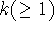married couples in the country. Then the language L = (Pr, Sp, T) adequate for this puzzle will be:where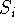denotes ith husband,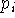means that's wife is unfaithful and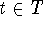denotes ith day.

Let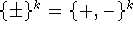denote that k-fold cartesian product of the vector space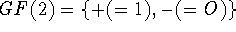with addition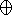. We define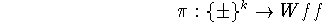by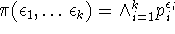, where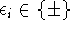and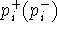denotes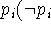, resp.). We put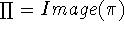and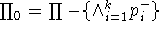. We also use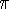to denote arbitrary element in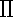. Now, let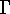denote what the King publicized on the first day, and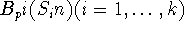denote a knowledge base for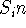under the situation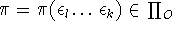. Let us put: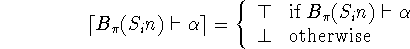and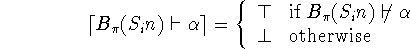where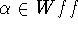. We also put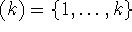. Then, as a formalization of the puzzle, we postulate the following identities: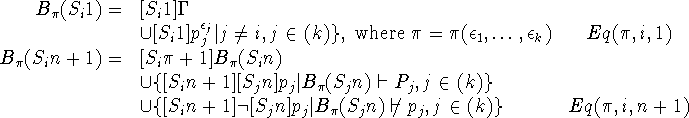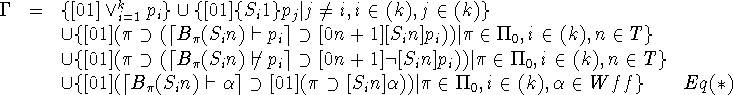Since the meta-notions such as knowledge base and provability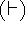cannot be expressed directly in our language, we were forced to interpret the King's decree intoin a somewhat indirect fashion.

Now, if we read Eq(*) as the definition of, then we find that the definition is circular, since in order thatmay be definable by Eq(*) it is necessary that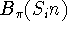are already defined, whereasare defined in terms ofin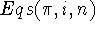. So, we will treat these equations as a system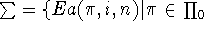,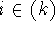,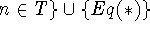of equations with the unknowns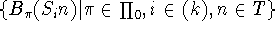and. We will solve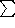under the following conditions:

(*) For any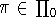,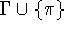is consistent.

(**) For anyand,is a knowledge base for.

We think these conditions are natural in view of the intended meanings ofand.

Let us define a norm on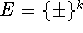by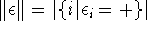, where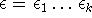. For any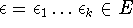and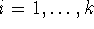, we put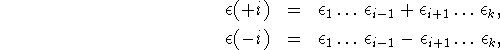and for any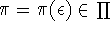, we put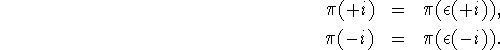We also put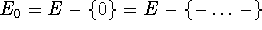.

We define a KT5-model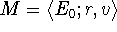as follows: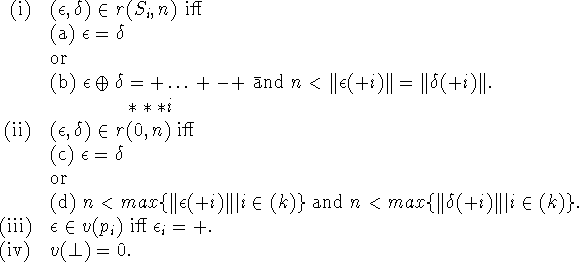Then we have the following theorem.

Theorem 8. Under the conditions () and (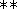),has the unique solution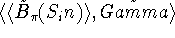, where the solution is characterized by the condition: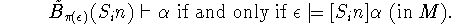Thus we have seen that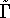may be regarded as the formal counterpart of the King's decree in our formal system. The puzzle is then reduced to the problem of showing that: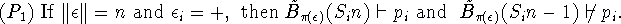We note that we can moreover prove the following: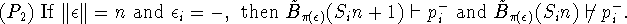It is clear that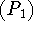and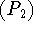follow at once from the condition stated in theorem 8.Next: References Up: The Puzzle of Unfaithful Previous: Informal presentation of the

Yasuko Kitajima
Fri Jun 20 13:39:43 PDT 1997